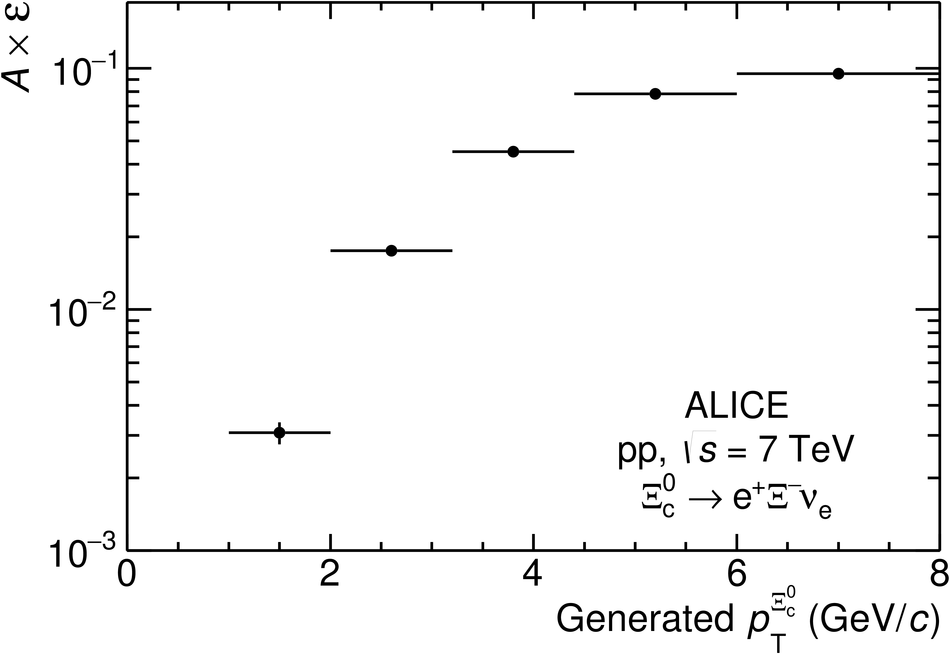# Figure 3

 Product of acceptance and efficiency ($A\times\varepsilon$) of \xicz\ baryons generated in $|y|< 0.8$ decaying into ${\rm e}^+\Xi^-\nu_{\rm e}$ as a function of \pt, determined from simulations {\sc pythia} 6 (see text).